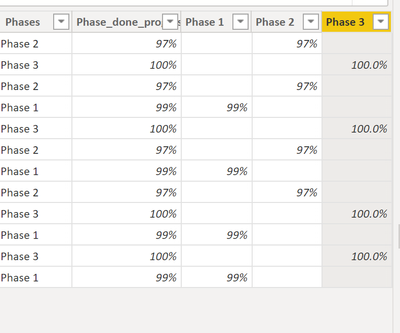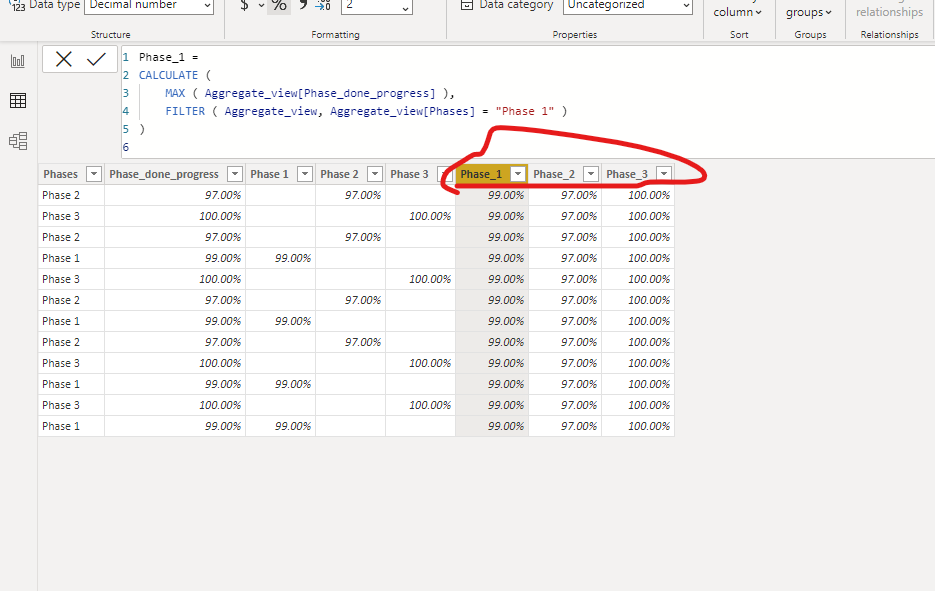cancel
Showing results for
Did you mean:Frequent Visitor

## Dax: Row to Column Transposition

Hi PowerBI users,

I would like to find out whether is possible to transpose rows into columns against Phases field. I have already prepared a intial logic however I still missing to populate the rest of the records which basically they do not meet the conditions :So in the end I would like repeat blank values with the same value even though it is not a correnspoding phase. The logic for Phase 1 for example is :

Phase 1 =

var Phase_1_value = if(Aggregate_view[Phases]="Phase 1",(Aggregate_view[Phase_done_progress]),BLANK())

return
Phase_1_value

Is there any way instead of having BLANK put the same result according to the PHASE ?

P.S. I know that there is a default transpose function in query and I am enforced to do in DAX.

1 ACCEPTED SOLUTIONCommunity Support

Hi, @Jaromac

You don't need to use 'if' statement at all. To replace the blank, you just need to change the code as below:

Calculated columns:

``````Phase_1 =
CALCULATE (
MAX ( Aggregate_view[Phase_done_progress] ),
FILTER ( Aggregate_view, Aggregate_view[Phases] = "Phase 1" )
)
``````
``````Phase_2 =
CALCULATE (
MAX ( Aggregate_view[Phase_done_progress] ),
FILTER ( Aggregate_view, Aggregate_view[Phases] = "Phase 2" )
)``````
``````Phase_3 =
CALCULATE (
MAX ( Aggregate_view[Phase_done_progress] ),
FILTER ( Aggregate_view, Aggregate_view[Phases] = "Phase 3" )
)``````Best Regards,
Community Support Team _ Eason

3 REPLIES 3Super User

Are those "Phase_done_progress" numbers always the same for each phase?

For instance, the Phase 2 is always 97%?Frequent Visitor

Yes 🙂 In the end I have a another column which reduce those numbers of records to have only one value but firstly I need to populate all blank records.Community Support

Hi, @Jaromac

You don't need to use 'if' statement at all. To replace the blank, you just need to change the code as below:

Calculated columns:

``````Phase_1 =
CALCULATE (
MAX ( Aggregate_view[Phase_done_progress] ),
FILTER ( Aggregate_view, Aggregate_view[Phases] = "Phase 1" )
)
``````
``````Phase_2 =
CALCULATE (
MAX ( Aggregate_view[Phase_done_progress] ),
FILTER ( Aggregate_view, Aggregate_view[Phases] = "Phase 2" )
)``````
``````Phase_3 =
CALCULATE (
MAX ( Aggregate_view[Phase_done_progress] ),
FILTER ( Aggregate_view, Aggregate_view[Phases] = "Phase 3" )
)``````Best Regards,
Community Support Team _ Eason